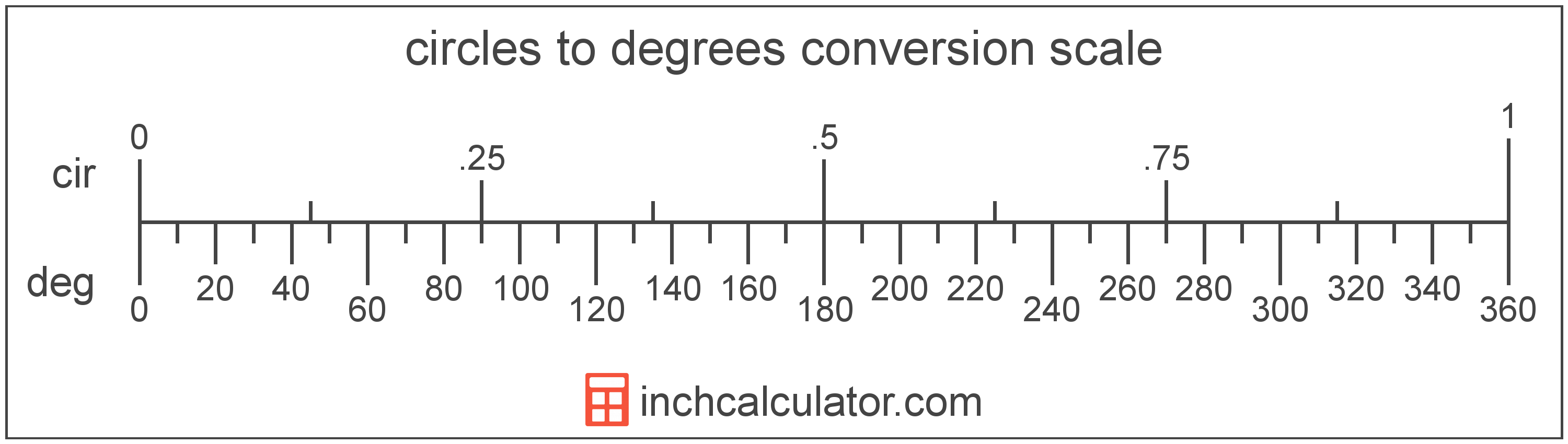# Circles to Degrees Conversion

Enter the angle in circles below to get the value converted to degrees.

Results in Degrees:1 cir = 360°
1 cir = 360° 0′ 0″
Do you want to convert degrees to circles?

## How to Convert Circles to DegreesTo convert a circle measurement to a degree measurement, multiply the angle by the conversion ratio.

Since one circle is equal to 360 degrees, you can use this simple formula to convert:

degrees = circles × 360

The angle in degrees is equal to the circles multiplied by 360.

For example, here's how to convert 5 circles to degrees using the formula above.
5 cir = (5 × 360) = 1,800°

### How Many Degrees are in a Circle?

There are 360 degrees in a circle, which is why we use this value in the formula above.

1 cir = 360°

## Circles

A circle is the equivalent of 1 revolution around a circle, or 360°.

A circle is sometimes also referred to as a revolution. Circles can be abbreviated as cir; for example, 1 circle can be written as 1 cir.

## Degrees

A degree is a measure of angle equal to 1/360th of a revolution, or circle. The number 360 has 24 divisors, making it a fairly easy number to work with. There are also 360 days in the Persian calendar year, and many theorize that early astronomers used 1 degree per day.

The degree is an SI accepted unit for angle for use with the metric system. A degree is sometimes also referred to as a degree of arc, arc degree, or arcdegree. Degrees can be abbreviated as °, and are also sometimes abbreviated as deg. For example, 1 degree can be written as 1° or 1 deg.

Degrees can also be expressed using minutes and seconds as an alternative to using the decimal form. Minutes and seconds are expressed using the prime (′) and double-prime (″) characters, although a single-quote and double-quote are often used for convenience.

One minute is equal to 1/60th of a degree, and one second is equal to 1/60th of a minute.

Protractors are commonly used to measure angles in degrees. They are semi-circle or full-circle devices with degree markings allowing a user to measure an angle in degrees. Learn more about how to use a protractor or download a printable protractor.

## Circle to Degree Conversion Table

Circle measurements converted to degrees
Circles Degrees
1 cir 360°
2 cir 720°
3 cir 1,080°
4 cir 1,440°
5 cir 1,800°
6 cir 2,160°
7 cir 2,520°
8 cir 2,880°
9 cir 3,240°
10 cir 3,600°
11 cir 3,960°
12 cir 4,320°
13 cir 4,680°
14 cir 5,040°
15 cir 5,400°
16 cir 5,760°
17 cir 6,120°
18 cir 6,480°
19 cir 6,840°
20 cir 7,200°
21 cir 7,560°
22 cir 7,920°
23 cir 8,280°
24 cir 8,640°
25 cir 9,000°
26 cir 9,360°
27 cir 9,720°
28 cir 10,080°
29 cir 10,440°
30 cir 10,800°
31 cir 11,160°
32 cir 11,520°
33 cir 11,880°
34 cir 12,240°
35 cir 12,600°
36 cir 12,960°
37 cir 13,320°
38 cir 13,680°
39 cir 14,040°
40 cir 14,400°

## References

1. Collins Dictionary, Definition of 'degree', https://www.collinsdictionary.com/us/dictionary/english/degree